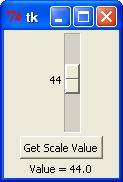# Python 3 - Tkinter Scale

The Scale widget provides a graphical slider object that allows you to select values from a specific scale.

## Syntax

Here is the simple syntax to create this widget −

``` w = Scale ( master, option, ... )
```

## Parameters

• master − This represents the parent window.

• options − Here is the list of most commonly used options for this widget. These options can be used as key-value pairs separated by commas.

Sr.No.Option & Description
1

activebackground

The background color when the mouse is over the scale.

2

bg

The background color of the parts of the widget that are outside the trough.

3

bd

Width of the 3-d border around the trough and slider. Default is 2 pixels.

4

command

A procedure to be called every time the slider is moved. This procedure will be passed one argument, the new scale value. If the slider is moved rapidly, you may not get a callback for every possible position, but you'll certainly get a callback when it settles.

5

cursor

If you set this option to a cursor name (arrow, dot etc.), the mouse cursor will change to that pattern when it is over the scale.

6

digits

The way your program reads the current value shown in a scale widget is through a control variable. The control variable for a scale can be an IntVar, a DoubleVar (float), or a StringVar. If it is a string variable, the digits option controls how many digits to use when the numeric scale value is converted to a string.

7

font

The font used for the label and annotations.

8

fg

The color of the text used for the label and annotations.

9

from_

A float or integer value that defines one end of the scale's range.

10

highlightbackground

The color of the focus highlight when the scale does not have focus.

11

highlightcolor

The color of the focus highlight when the scale has the focus.

12

label

You can display a label within the scale widget by setting this option to the label's text. The label appears in the top left corner if the scale is horizontal, or the top right corner if vertical. The default is no label.

13

length

The length of the scale widget. This is the x dimension if the scale is horizontal, or the y dimension if vertical. The default is 100 pixels.

14

orient

Set orient = HORIZONTAL if you want the scale to run along the x dimension, or orient = VERTICAL to run parallel to the y-axis. Default is horizontal.

15

relief

Specifies the appearance of a decorative border around the label. The default is FLAT; for other values.

16

repeatdelay

This option controls how long button 1 has to be held down in the trough before the slider starts moving in that direction repeatedly. Default is repeatdelay = 300, and the units are milliseconds.

17

resolution

Normally, the user will only be able to change the scale in whole units. Set this option to some other value to change the smallest increment of the scale's value. For example, if from_ = -1.0 and to = 1.0, and you set resolution = 0.5, the scale will have 5 possible values: -1.0, -0.5, 0.0, +0.5, and +1.0.

18

showvalue

Normally, the current value of the scale is displayed in text form by the slider (above it for horizontal scales, to the left for vertical scales). Set this option to 0 to suppress that label.

19

sliderlength

Normally the slider is 30 pixels along the length of the scale. You can change that length by setting the sliderlength option to your desired length.

20

state

Normally, scale widgets respond to mouse events, and when they have the focus, also keyboard events. Set state = DISABLED to make the widget unresponsive.

21

takefocus

Normally, the focus will cycle through scale widgets. Set this option to 0 if you don't want this behavior.

22

tickinterval

To display periodic scale values, set this option to a number, and ticks will be displayed on multiples of that value. For example, if from_ = 0.0, to = 1.0, and tickinterval = 0.25, labels will be displayed along the scale at values 0.0, 0.25, 0.50, 0.75, and 1.00. These labels appear below the scale if horizontal, to its left if vertical. Default is 0, which suppresses display of ticks.

23

to

A float or integer value that defines one end of the scale's range; the other end is defined by the from_ option, discussed above. The to value can be either greater than or less than the from_ value. For vertical scales, the to value defines the bottom of the scale; for horizontal scales, the right end.

24

troughcolor

The color of the trough.

25

variable

The control variable for this scale, if any. Control variables may be from class IntVar, DoubleVar (float), or StringVar. In the latter case, the numerical value will be converted to a string.

26

width

The width of the trough part of the widget. This is the x dimension for vertical scales and the y dimension if the scale has orient = HORIZONTAL. Default is 15 pixels.

## Methods

Scale objects have these methods −

Sr.No.Method & Description
get()This method returns the current value of the scale.
set ( value )Sets the scale's value.

## Example

Try the following example yourself −

```# !/usr/bin/python3
from tkinter import *

def sel():
selection = "Value = " + str(var.get())
label.config(text = selection)

root = Tk()
var = DoubleVar()
scale = Scale( root, variable = var )
scale.pack(anchor = CENTER)

button = Button(root, text = "Get Scale Value", command = sel)
button.pack(anchor = CENTER)

label = Label(root)
label.pack()

root.mainloop()
```

## Result

When the above code is executed, it produces the following result −python_gui_programming.htm